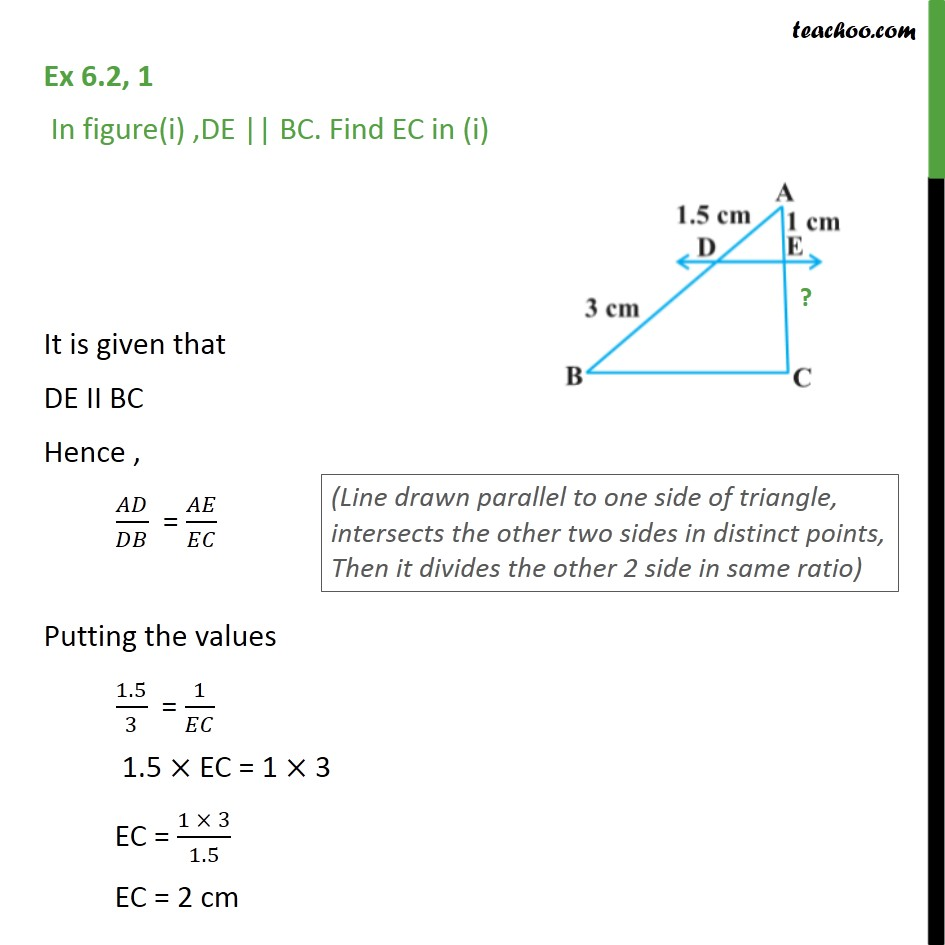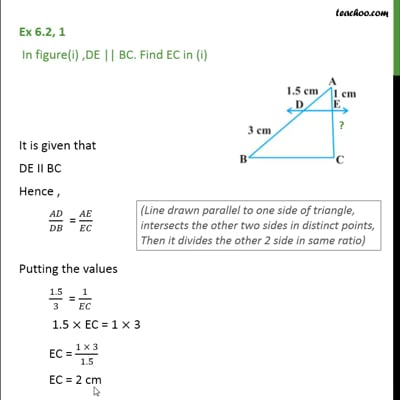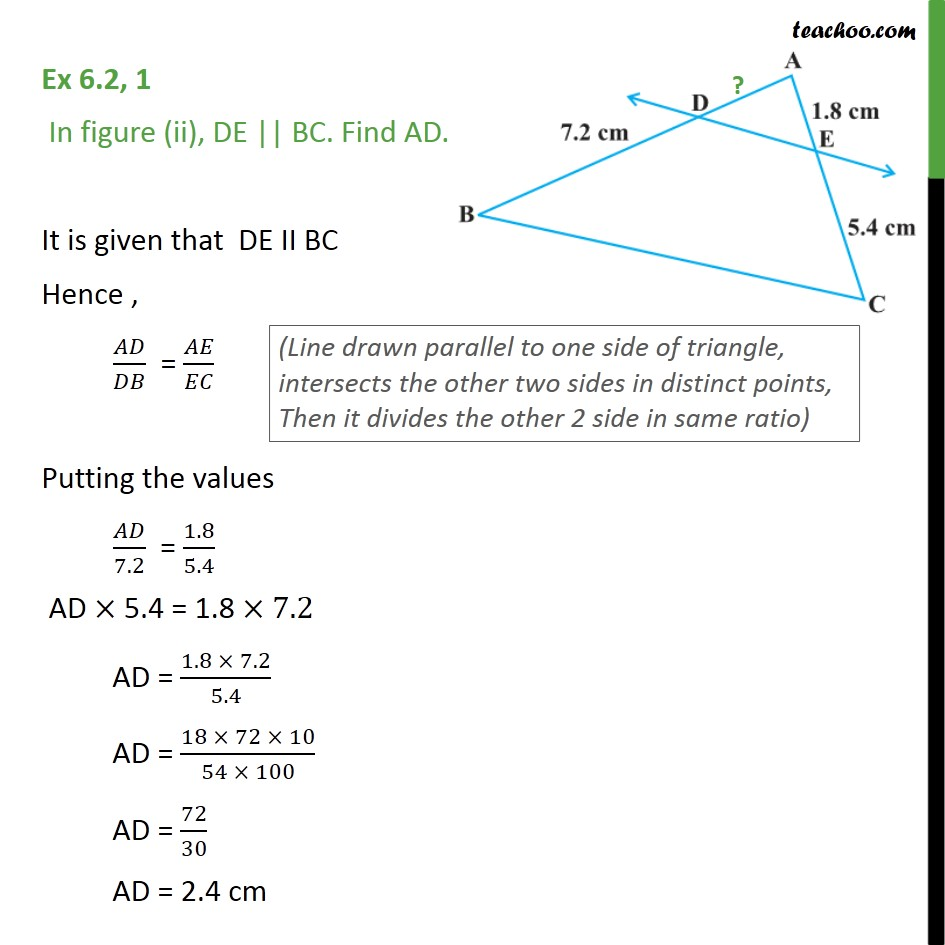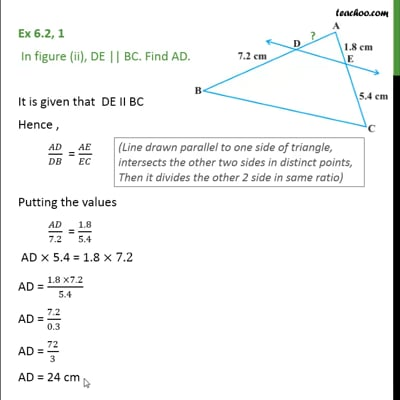Theorem 6.1

Chapter 6 Class 10 Triangles
Concept wiseThis video is only available for Teachoo black usersThis video is only available for Teachoo black users

Solve all your doubts with Teachoo Black (new monthly pack available now!)

### Transcript

Ex 6.2, 1 In figure(i) ,DE || BC. Find EC in (i) It is given that DE II BC Hence , / = / Putting the values 1.5/3 = 1/ 1.5 EC = 1 3 EC = (1 3)/1.5 EC = 2 cm Ex 6.2, 1 In figure (ii), DE || BC. Find AD. It is given that DE II BC Hence , 𝐴𝐷/𝐷𝐵 = 𝐴𝐸/𝐸𝐶 Putting the values 𝐴𝐷/7.2 = 1.8/5.4 AD × 5.4 = 1.8 ×7.2 AD = (1.8 × 7.2)/5.4 AD = (18 × 72 × 10)/(54 × 100) AD = 72/30 AD = 2.4 cm